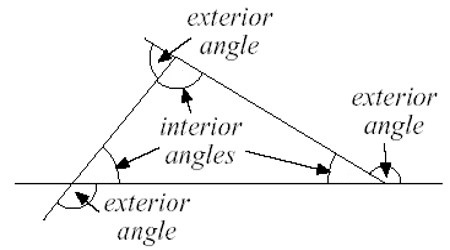Triangle is a plane figure with three straight sides and three angles. The three angles always add to 180°. The vertex is a corner of the triangle. Every triangle has three vertices. The interior angles of a triangle always add up to 180°. The exterior angles of a triangle always add up to 360°.

Types of triangles based on their angles

• A RIGHT triangle has one 900
• An OBTUSE triangle has one angle that is greater than 900.
• An ACUTE triangle has all three angles less than 900.Fig: Angles of a Triangle

Properties of Angles of a Triangle

1. The three angles of a triangle are together equal to two right angles.

ABC is a triangle.

Then ∠ZXY + ∠XYZ + ∠YZX = 180°

Using this property, let us solve some of the examples.

Solved examples:

(i) In ∆XYZ, ∠X = 55° and ∠Y = 75°. Find ∠Z.

Solution:

∠X + ∠Y + ∠Z = 180°

or, 55° + 75° + ∠Z = 180°

or, 130° + ∠Z = 180°

or, 130° – 130° + ∠Z = 180° – 130°

Therefore, ∠Z = 50°

(ii) In the ∆XYZ, ∠Y = 5∠Z and ∠X= 3∠Z. Find the angles of the triangle.

Solution:

∠X + ∠Y + ∠Z = 180°

or, 3∠Z + 5∠Z + ∠Z = 180°

or, 9∠Z = 180°

or, 9∠Z / 9 = 180°/9

Therefore, ∠Z = 20°

We know, ∠X= 3∠Z

Now, plug-in the value of ∠Z

∠X= 3 × 20°

Therefore, ∠X= 60°

Again we know, ∠Y= 5∠Z

Now, plug-in the value of ∠Z

∠Y= 5 × 20°

Therefore, ∠Y= 100°

Hence, the angles of the triangle are ∠X = 60°, ∠Y = 100° and ∠Z = 20°.

1. If one side of a triangle is produced, the exterior angle so formed is equal to the sum of the two interior opposite angles.

The side QR of the ∆PQR is produced to S.

Then ∠PRS = ∠RPQ + ∠PQR

Corollary 1: An exterior angle of a triangle is greater than either of the interior opposite angles.

In ∆PQR, QR is produced to S.

Therefore, ∠PRS > ∠RPQ and ∠PRS ∠PQR

Corollary 2: A triangle can have only one right angle.

Corollary 3: A triangle can have only one obtuse angle.

Corollary 4: A triangle must have at least two acute angles.

Corollary 5: In a right-angled triangle, the acute angles are complementary.

Information Source: